# What is the most efficient way to trim time from datetime?

SentryOne eBooks

In these books, you will find useful, hand-picked articles that will help give insight into some of your most vexing performance problems. These articles were written by several of the SQL Server industry’s leading experts, including Paul White, Paul Randal, Jonathan Kehayias, Erin Stellato, Glenn Berry, Aaron Bertrand, and Joe Sack.

Featured AuthorErin Stellato is a Principal Consultant with SQLskills and a Microsoft Data Platform MVP.

Erin’s Posts

The most common need for stripping time from a datetime value is to get all the rows that represent orders (or visits, or accidents) that occurred on a given day. However, not all of the techniques that are used to do so are efficient or even safe.

## TL;DR version

If you want a safe range query that performs well, use an open-ended range or, for single-day queries on SQL Server 2008 and above, use `CONVERT(DATE)`:

```DECLARE @today DATETIME;

-- only on <= 2005:

SET @today = DATEADD(DAY, DATEDIFF(DAY, '20000101', CURRENT_TIMESTAMP), '20000101');

-- or on 2008 and above:

SET @today = CONVERT(DATE, CURRENT_TIMESTAMP);

-- and then use an open-ended range in the query:

...
WHERE OrderDate >= @today
AND OrderDate < DATEADD(DAY, 1, @today);

-- you can also do this (again, in SQL Server 2008 and above):

...
WHERE CONVERT(DATE, OrderDate) = @today;```

Some caveats:

• Be careful with the `DATEDIFF` approach, as there are some cardinality estimation anomalies that can occur (see this blog post and the Stack Overflow question that spurred it for more information).
• While the last one will still potentially use an index seek (unlike every other non-sargable expression I've ever come across), you need to be careful about converting the column to a date before comparing. This approach, too, can yield fundamentally wrong cardinality estimations. See this answer by Martin Smith for more details.

In any case, read on to understand why these are the only two approaches I ever recommend.

## Not all approaches are safe

As an unsafe example, I see this one used a lot:

```WHERE OrderDate BETWEEN DATEADD(DAY, DATEDIFF(DAY, 0, GETDATE()), 0)

There are a few problems with this approach, but the most notable one is the calculation of the "end" of today – if the underlying data type is `SMALLDATETIME`, that end range is going to round up; if it is `DATETIME2`, you could theoretically miss data at the end of the day. If you pick minutes or nanoseconds or any other gap to accommodate the current data type, your query will start having different behavior should the data type ever change later (and let's be honest, if someone changes that column's type to be more or less granular, they're not running around checking every single query that accesses it). Having to code this way depending on the type of date/time data in the underlying column is fragmented and error-prone. It is much better to use open-ended date ranges for this:

```WHERE OrderDate >= CONVERT(date, GETDATE())
AND OrderDate < DATEADD(DAY, 1, CONVERT(date, GETDATE()));```

But I wanted to compare the performance of some of the more common approaches I see out there. I've long used open-ended ranges exclusively, and since SQL Server 2008 we've been able to use `CONVERT(DATE)` and still utilize an index on that column, which is quite powerful.

```SELECT CONVERT(CHAR(8), CURRENT_TIMESTAMP, 112);
SELECT CONVERT(CHAR(10), CURRENT_TIMESTAMP, 120);
SELECT CONVERT(DATE, CURRENT_TIMESTAMP);
SELECT DATEADD(DAY, DATEDIFF(DAY, '19000101', CURRENT_TIMESTAMP), '19000101');
SELECT CONVERT(DATETIME, DATEDIFF(DAY, '19000101', CURRENT_TIMESTAMP));
SELECT CONVERT(DATETIME, CONVERT(INT, CONVERT(FLOAT, CURRENT_TIMESTAMP)));
SELECT CONVERT(DATETIME, FLOOR(CONVERT(FLOAT, CURRENT_TIMESTAMP)));```

## A Simple Performance Test

To perform a very simple initial performance test, I did the following for each of the above statements, setting a variable to the output of the calculation 100,000 times:

```SELECT SYSDATETIME();
GO

DECLARE @d DATETIME = [conversion method];
GO 100000

SELECT SYSDATETIME();
GO```

I did this three times for each method, and they all ran in the range of 34-38 seconds. So strictly speaking, there are very negligible differences in these methods when performing the operations in memory: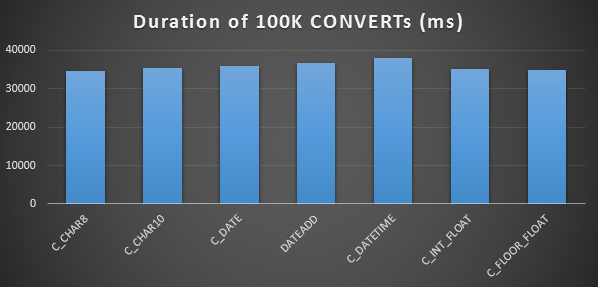## A More Elaborate Performance Test

I also wanted to compare these methods with different data types (`DATETIME`, `SMALLDATETIME`, and `DATETIME2`), against both a clustered index and a heap, and with and without data compression. So first I created a simple database. Through experimentation I determined that the optimal size to handle 120 million rows and all of the log activity that might incur (and to prevent auto-grow events from interfering with the testing) was a 20GB data file and a 3GB log:

```CREATE DATABASE [Datetime_Testing]
ON PRIMARY
(
NAME = N'Datetime_Testing_Data',
FILENAME = N'D:\DATA\Datetime_Testing.mdf',
SIZE = 20480000KB , MAXSIZE = UNLIMITED, FILEGROWTH = 102400KB
)
LOG ON
(
NAME = N'Datetime_Testing_Log',
FILENAME = N'E:\LOGS\Datetime_Testing_log.ldf',
SIZE = 3000000KB , MAXSIZE = UNLIMITED, FILEGROWTH = 20480KB );```

Next, I created 12 tables:

```-- clustered index with no compression:

CREATE TABLE dbo.smalldatetime_nocompression_clustered(dt SMALLDATETIME);
CREATE CLUSTERED INDEX x ON dbo.smalldatetime_nocompression_clustered(dt);

-- heap with no compression:

CREATE TABLE dbo.smalldatetime_nocompression_heap(dt SMALLDATETIME);

-- clustered index with page compression:

CREATE TABLE dbo.smalldatetime_compression_clustered(dt SMALLDATETIME)
WITH (DATA_COMPRESSION = PAGE);

CREATE CLUSTERED INDEX x ON dbo.smalldatetime_compression_clustered(dt)
WITH (DATA_COMPRESSION = PAGE);

-- heap with page compression:

CREATE TABLE dbo.smalldatetime_compression_heap(dt SMALLDATETIME)
WITH (DATA_COMPRESSION = PAGE);```

[Then repeat again for DATETIME and DATETIME2.]

Next, I inserted 10,000,000 rows into each table. I did this by creating a view that would generate the same 10,000,000 dates each time:

```CREATE VIEW dbo.TenMillionDates
AS
SELECT TOP (10000000) d = DATEADD(MINUTE, ROW_NUMBER() OVER
(ORDER BY s1.[object_id]), '19700101')
FROM sys.all_columns AS s1
CROSS JOIN sys.all_objects AS s2
ORDER BY s1.[object_id];```

This allowed me to populate the tables this way:

```INSERT /* dt_comp_clus */ dbo.datetime_compression_clustered(dt)
SELECT CONVERT(DATETIME, d) FROM dbo.TenMillionDates;
CHECKPOINT;
INSERT /* dt2_comp_clus */ dbo.datetime2_compression_clustered(dt)
SELECT CONVERT(DATETIME2, d) FROM dbo.TenMillionDates;
CHECKPOINT;
INSERT /* sdt_comp_clus */ dbo.smalldatetime_compression_clustered(dt)
SELECT CONVERT(SMALLDATETIME, d) FROM dbo.TenMillionDates;
CHECKPOINT;```

[Then repeat again for the heaps and the non-compressed clustered index. I put a `CHECKPOINT` between each insert to ensure log reuse (the recovery model is simple).]

## INSERT Timings & Space Used

Here are the timings for each insert (as captured with Plan Explorer):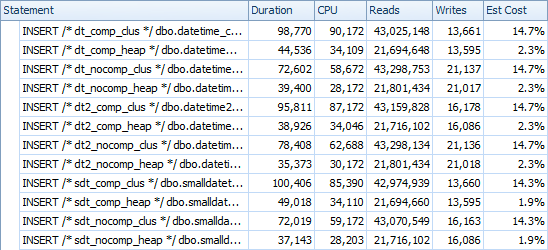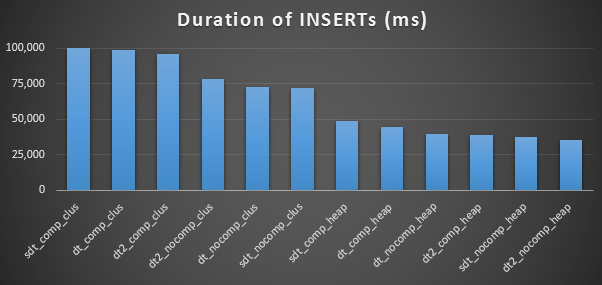And here is the amount of space occupied by each table:

```SELECT
[table] = OBJECT_NAME([object_id]),
row_count,
page_count = reserved_page_count,
reserved_size_MB = reserved_page_count * 8/1024
FROM sys.dm_db_partition_stats
WHERE OBJECT_NAME([object_id]) LIKE '%datetime%';```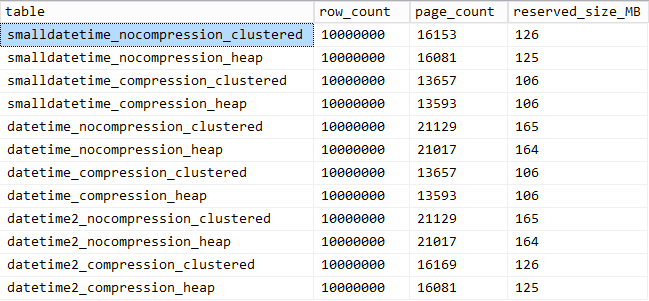## Query Pattern Performance

Next I set out to test two different query patterns for performance:

• Counting the rows for a specific day, using the above seven approaches, as well as the open-ended date range
• Converting all 10,000,000 rows using the above seven approaches, as well as just returning the raw data (since formatting on the client side may be better)

[With the exception of the `FLOAT` methods and the `DATETIME2` column, since this conversion is not legal.]

For the first question, the queries look like this (repeated for each table type):

```SELECT /* C_CHAR10 - dt_comp_clus */ COUNT(*)
FROM dbo.datetime_compression_clustered
WHERE CONVERT(CHAR(10), dt, 120) = '19860301';

SELECT /* C_CHAR8  - dt_comp_clus */ COUNT(*)
FROM dbo.datetime_compression_clustered
WHERE CONVERT(CHAR(8),  dt, 112) = '19860301';

SELECT /* C_FLOOR_FLOAT - dt_comp_clus */ COUNT(*)
FROM dbo.datetime_compression_clustered
WHERE CONVERT(DATETIME, FLOOR(CONVERT(FLOAT, dt))) = '19860301';

SELECT /* C_DATETIME  - dt_comp_clus */ COUNT(*)
FROM dbo.datetime_compression_clustered
WHERE CONVERT(DATETIME, DATEDIFF(DAY, '19000101', dt)) = '19860301';

SELECT /* C_DATE  - dt_comp_clus */ COUNT(*)
FROM dbo.datetime_compression_clustered
WHERE CONVERT(DATE, dt) = '19860301';

SELECT /* C_INT_FLOAT - dt_comp_clus */ COUNT(*)
FROM dbo.datetime_compression_clustered
WHERE CONVERT(DATETIME, CONVERT(INT, CONVERT(FLOAT, dt))) = '19860301';

SELECT /* DATEADD - dt_comp_clus */ COUNT(*)
FROM dbo.datetime_compression_clustered
WHERE DATEADD(DAY, DATEDIFF(DAY, '19000101', dt), '19000101') = '19860301';

SELECT /* RANGE - dt_comp_clus */ COUNT(*)
FROM dbo.datetime_compression_clustered
WHERE dt >= '19860301' AND dt < '19860302';```

The results against a clustered index look like this (click to enlarge):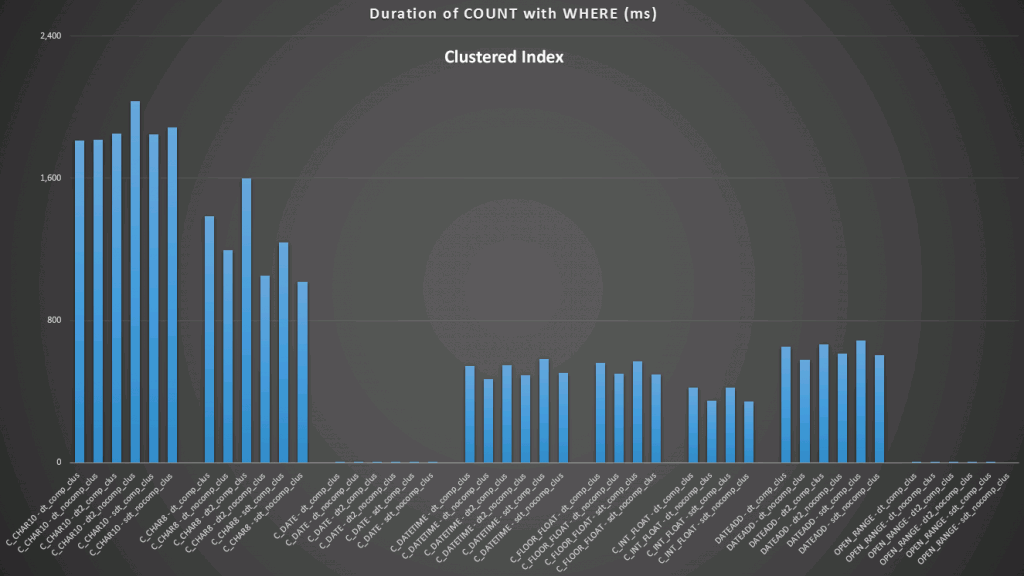Here we see that the convert to date and the open-ended range using an index are the best performers. However, against a heap, the convert to date actually takes some time, making the open-ended range the optimal choice (click to enlarge):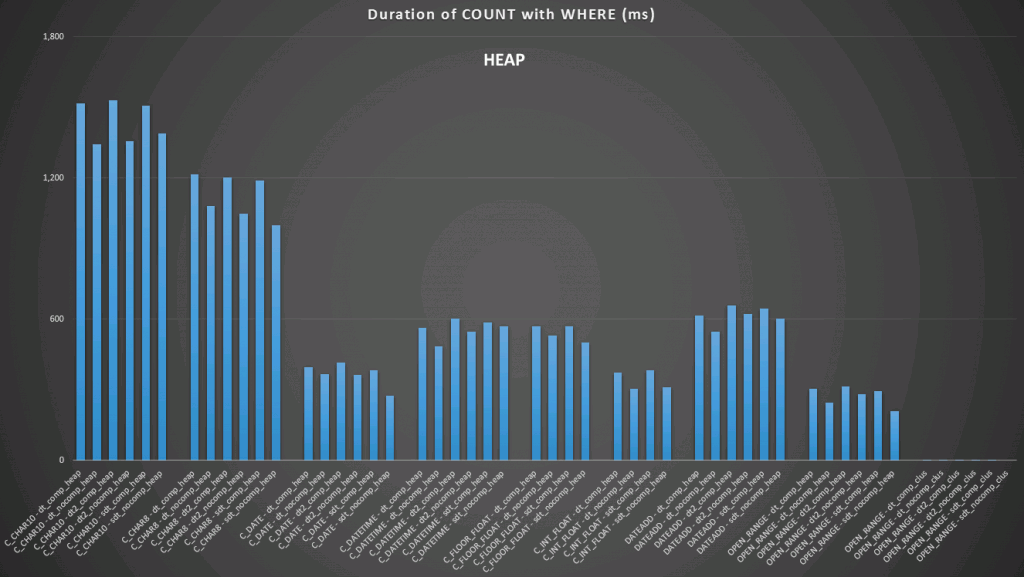And here are the second set of queries (again, repeating for each table type):

```SELECT /* C_CHAR10 - dt_comp_clus */ dt = CONVERT(CHAR(10), dt, 120)
FROM dbo.datetime_compression_clustered;

SELECT /* C_CHAR8 - dt_comp_clus */ dt = CONVERT(CHAR(8), dt, 112)
FROM dbo.datetime_compression_clustered;

SELECT /* C_FLOOR_FLOAT - dt_comp_clus */ dt = CONVERT(DATETIME, FLOOR(CONVERT(FLOAT, dt)))
FROM dbo.datetime_compression_clustered;

SELECT /* C_DATETIME  - dt_comp_clus */ dt = CONVERT(DATETIME, DATEDIFF(DAY, '19000101', dt))
FROM dbo.datetime_compression_clustered;

SELECT /* C_DATE  - dt_comp_clus */ dt = CONVERT(DATE, dt)
FROM dbo.datetime_compression_clustered;

SELECT /* C_INT_FLOAT - dt_comp_clus */ dt = CONVERT(DATETIME, CONVERT(INT, CONVERT(FLOAT, dt)))
FROM dbo.datetime_compression_clustered;

SELECT /* DATEADD - dt_comp_clus */ dt = DATEADD(DAY, DATEDIFF(DAY, '19000101', dt), '19000101')
FROM dbo.datetime_compression_clustered;

SELECT /* RAW - dt_comp_clus */ dt
FROM dbo.datetime_compression_clustered;```

Focusing on the results for tables with a clustered index, it is clear that the convert to date was a very close performer to just selecting the raw data (click to enlarge):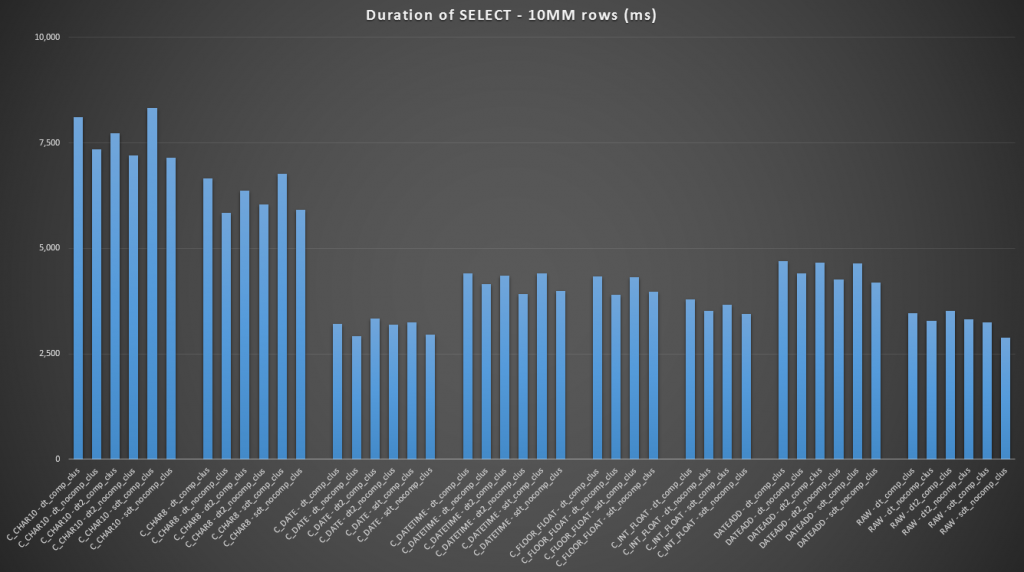(For this set of queries, the heap showed very similar results – practically indistinguishable.)

## Conclusion

In case you wanted to skip to the punchline, these results show that conversions in memory are not important, but if you are converting data on the way out of a table (or as part of a search predicate), the method you choose can have a dramatic impact on performance. Converting to a `DATE` (for a single day) or using an open-ended date range in any case will yield the best performance, while the most popular method out there – converting to a string – is absolutely abysmal.

We also see that compression can have a decent effect on storage space, with very minor impact on query performance. The effect on insert performance seems to be as dependent on whether or not the table has a clustered index rather than whether or not compression is enabled. However, with a clustered index in place, there was a noticeable bump in the duration it took to insert 10 million rows. Something to keep in mind and to balance with disk space savings.

Clearly there could be a lot more testing involved, with more substantial and varied workloads, which I may explore further in a future post.